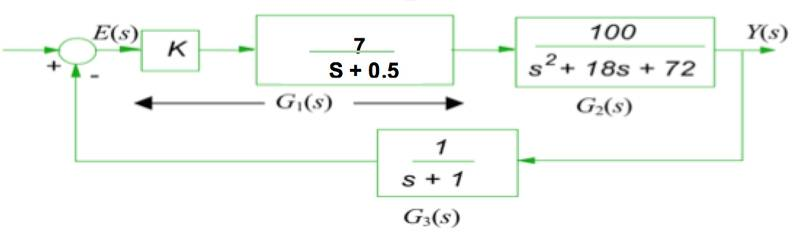# Calculating Steady State Error

dontigeh
I am trying to calculate the steady state error of the following system but unable to do it. I have used MATLAB and calculated the steady state error to be 0.1128 but don't understand the steps that I need to do to calculate this.

Thanks## Answers and Replies

milesyoung
I am trying to calculate the steady state error of the following system but unable to do it. I have used MATLAB and calculated the steady state error to be 0.1128 but don't understand the steps that I need to do to calculate this.

Do you mean the steady-state error to a step input?

What value of ##K## did you use? The system is unstable for ##K = 1##.

In general, you could find the transfer function from the input to ##E(s)##, verify it's stable, and use the final value theorem.

•timthereaper
dontigeh
Do you mean the steady-state error to a step input?

What value of ##K## did you use? The system is unstable for ##K = 1##.

In general, you could find the transfer function from the input to ##E(s)##, verify it's stable, and use the final value theorem.

Yes I want the steady-state error to a step input.

And the value of K used was 0.375.

milesyoung
Yes I want the steady-state error to a step input.

And the value of K used was 0.375.
Right, so you could use the general method I described, or if you've had a lecture on error constants, you could calculate that instead.

timthereaper
I agree with @milesyoung and say you should compute the closed-loop transfer function and use the final value theorem.

dontigeh
Hi,
Thanks for the help.
I did the following: 1/s(1-(262.5s+262.5)+(700/0.375S^4+7.313s^3+37.313s^2+43.875s+276)), then i did E(infinity) = lim s-> 0 [ 1-700/736]= 0.04891. Which is not correct. The gain is 0.375.

milesyoung
Hi,
Thanks for the help.
I did the following: 1/s(1-(262.5s+262.5)+(700/0.375S^4+7.313s^3+37.313s^2+43.875s+276)), then i did E(infinity) = lim s-> 0 [ 1-700/736]= 0.04891. Which is not correct. The gain is 0.375.
I can't decipher what went wrong if you don't show more detail.

I'd suggest you don't multiply anything out. Just find ##E(s)## symbolically using ##G_1(s),G_2(s),G_3(s)##, and then take the limit.

Gold Member
Since you are going to take the limit of the final formula as s->0, you can do that in each part as the first step. That will simplify things tremendously.
That gives a steady state gain of .375 * 7/0.5 * 100/72 = 7.29166666666667 across the top and a steady state gain of 1 for G3 in the feedback loop.
For the entire system I get a steady state gain of 7.29166666666667/(1+7.29166666666667) = 0.879396984924623.
For a unit step input, that would give a steady state error of 1-0.879396984924623 = 0.120603015075377.
I don't know why the MATLAB answer 0.1128 is different. Is it possible that the MATLAB number is from a finite time plot that is an approximation to infinite time?

Last edited:
•dontigeh and timthereaper